An intuitive library to plot evaluation metrics.

# kds - KeyToDataScience Visualization Library

### Just input 'labels' and 'probabilities' to get quick report for analysis

kds.metrics.report(y_test, y_prob)


Report has an argument plot_style which has multiple plot style options. For more, explore examples !!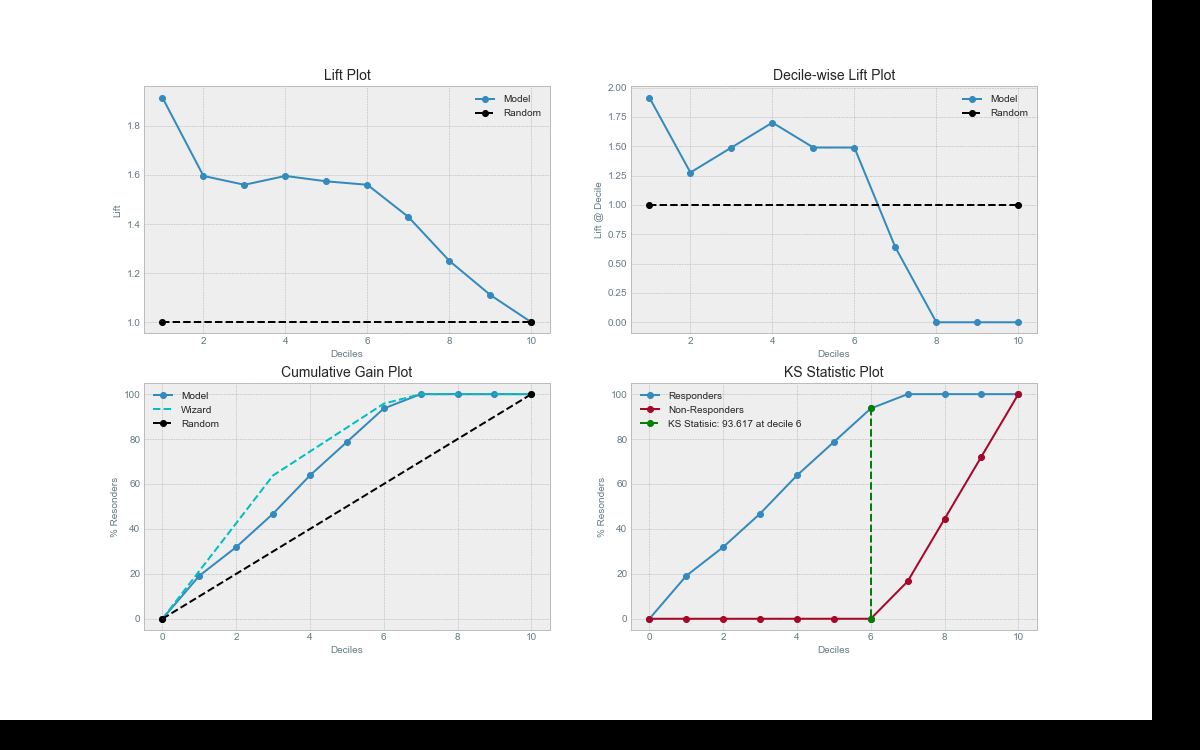kds is the result of a data scientist's humble effort to provide an easy way of visualizing metrics. So that one can focus on the analysis rather than hassling with copy/paste of various visialization functions.

## Installation

Installation is simple! Just double check, you have the dependencies Pandas, Numpy and Matplotlib installed.

Then just run:

pip install kds


Or if you want the latest development version, clone this repo and run

python setup.py install


at the root folder.

## Examples

Let's dive into using various plots with the sample iris dataset from scikit-learn.

### 1. Lift Plot

# REPRODUCABLE EXAMPLE
# Load Dataset and train-test split
from sklearn.model_selection import train_test_split
from sklearn import tree

X_train, X_test, y_train, y_test = train_test_split(X, y, test_size=0.33,random_state=3)
clf = tree.DecisionTreeClassifier(max_depth=1,random_state=3)
clf = clf.fit(X_train, y_train)
y_prob = clf.predict_proba(X_test)

# The magic happens here
import kds
kds.metrics.plot_lift(y_test, y_prob[:,1])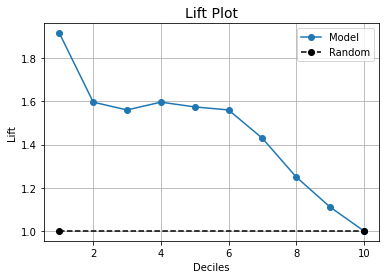Yup... That's it. single line functions for detailed visualization.

You can see clearly here that kds.metrics.lift needs only the actual y_true values and the predicted probabilities to generate the plot. This lets you use anything you want as the classifier, from Random Forest to Keras NNs to XgBoost to any classifier algorithm you want to use.

Want to see more exapmles ??

### 2. Cumulative Gain Plot

# REPRODUCABLE EXAMPLE
# Load Dataset and train-test split
from sklearn.model_selection import train_test_split
from sklearn import tree

X_train, X_test, y_train, y_test = train_test_split(X, y, test_size=0.33,random_state=3)
clf = tree.DecisionTreeClassifier(max_depth=1,random_state=3)
clf = clf.fit(X_train, y_train)
y_prob = clf.predict_proba(X_test)

# The magic happens here
import kds
kds.metrics.plot_cumulative_gain(y_test, y_prob[:,1])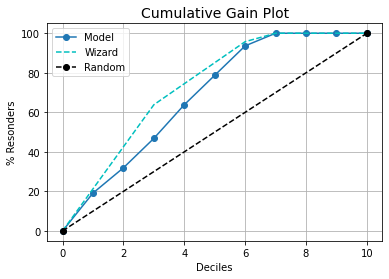### 3. KS Statistic Plot

# REPRODUCABLE EXAMPLE
# Load Dataset and train-test split
from sklearn.model_selection import train_test_split
from sklearn import tree

X_train, X_test, y_train, y_test = train_test_split(X, y, test_size=0.33,random_state=3)
clf = tree.DecisionTreeClassifier(max_depth=1,random_state=3)
clf = clf.fit(X_train, y_train)
y_prob = clf.predict_proba(X_test)

# The magic happens here
import kds
kds.metrics.plot_ks_statistic(y_test, y_prob[:,1])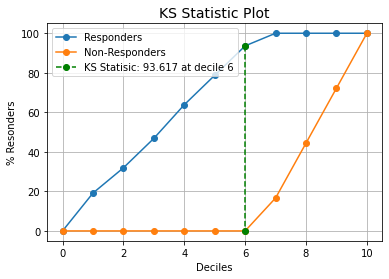### 4. Decile Table

# REPRODUCABLE EXAMPLE
# Load Dataset and train-test split
from sklearn.model_selection import train_test_split
from sklearn import tree

X_train, X_test, y_train, y_test = train_test_split(X, y, test_size=0.33,random_state=3)
clf = tree.DecisionTreeClassifier(max_depth=1,random_state=3)
clf = clf.fit(X_train, y_train)
y_prob = clf.predict_proba(X_test)

# The magic happens here
import kds
kds.metrics.decile_table(y_test, y_prob[:,1])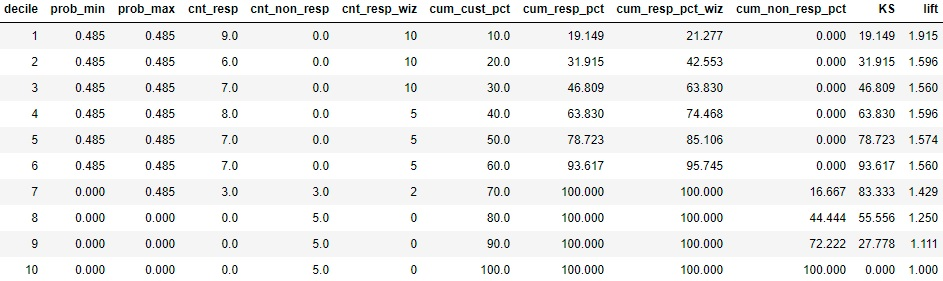### 5. Report

# REPRODUCABLE EXAMPLE
# Load Dataset and train-test split
from sklearn.model_selection import train_test_split
from sklearn import tree

X_train, X_test, y_train, y_test = train_test_split(X, y, test_size=0.33,random_state=3)
clf = tree.DecisionTreeClassifier(max_depth=1,random_state=3)
clf = clf.fit(X_train, y_train)
y_prob = clf.predict_proba(X_test)

# The magic happens here
import kds
kds.metrics.report(y_test, y_prob[:,1],plot_style='ggplot')


Choose among multiple plot_style list using plt.style.available, to generate quick and beautiful plots.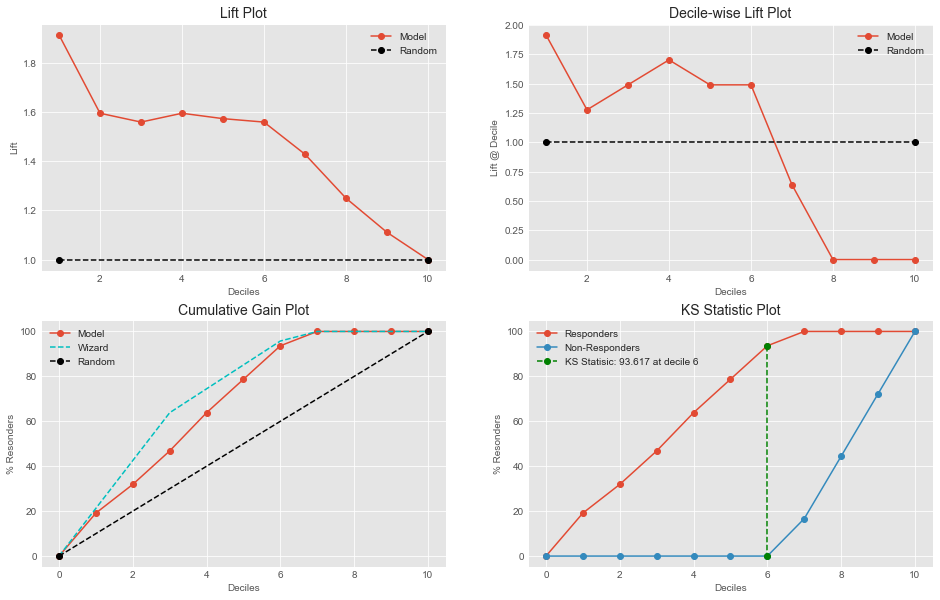## Contributing to kds

Contributions are what make the open source community such an amazing place to be learn, inspire, and create. Any contributions you make are greatly appreciated.

Please read CONTRIBUTING.md for details on our code of conduct, and the process for submitting pull requests to us. Visit our contributor guidelines.

Happy plotting!

Change Log

================

## 0.1.3 (15/06/2021)

• Add new parameter:'change_decile' in decile_table to change number of partitions. Updated bug in calling kds.metrics.report.

## 0.1.1 (27/01/2021)

• Updated Readme and plot styles

## 0.1.0 (27/01/2021)

• First Release

## Project details

This version0.1.30.1.20.1.10.1.0

Uploaded source
Uploaded py3